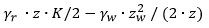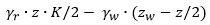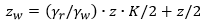The Rocscience International Conference 2021 Proceedings are now available. Read Now

# Gravity Lateral Stress

With this option, average lateral stresses are calculated based on the locked in stress, horizontal to vertical stress ratios, the pillar thickness, and the water height (if pillar is permeable) as follows:

Lateral stress = locked in stress + change in lateral stress

## Locked In Stress

This is the lateral stress at the surface of the pillar, and is assumed constant with depth. This value can be manipulated to represent preexisting stress conditions, or the effects of the overburden on the lateral stress.

## Change in Lateral Stress from Ground Surface to Mid-Height of Pillar

Change in lateral stress =where,If water height is > pillar thickness, the water pressure component of the above formula is modified accordingly.

Change in lateral stress =## Critical Water Height

Note that the above equation allows the calculation of the water height which will produce zero effective stress (if pillar is permeable) as shown in the equation below. If a water height greater than this is specified, negative effective stresses will result and calculation will not proceed, until the user acknowledges the warning prompt.

If no Locked In Stress is considered:

CriticalSince the vertical pressure is based only on the thickness of the pillar, it can be seen that this option is only strictly applicable for surface crown pillars. Only an average value of the lateral stress is calculated by the method, the true variation of field stress with depth is not considered. Therefore, the Gravity option as presented in CPillar is actually a type of Constant stress option, which calculates an average lateral stress based on the thickness of the pillar, and the user input horizontal / vertical stress ratios